# B.Tech. (SEM-II) THEORY EXAMINATION 2015-16 COMPUTER SYSTEM & PROGRAMMING IN C

Time: 3hrs
Total Marks: 100

Note:-

• There are three sections. Section A carries 20 marks, Section B carries 50 marks and Section C carries 30 marks.
• Attempt all questions. Marks are indicated against each question.
• Assume suitable data wherever necessary.

### Section – B

2. Attempt any five parts: (10*5 = 50)

1. What is special about void pointer?

A void pointer is a pointer that has no associated data type with it. A void pointer can hold address of any type and can be typcasted to any type.

 `int` `a = 10; ` `char` `b = ``'x'``; ` ` `  `void``* p = &a; ``// void pointer holds address of int 'a' ` `p = &b; ``// void pointer holds address of char 'b' `

1) malloc() and calloc() return void * type and this allows these functions to be used to allocate memory of any data type (just because of void *)

 `int main(void) ` `{ ` `    ``// Note that malloc() returns void * which can be ` `    ``// typecasted to any type like int *, char *, .. ` `    ``int* x = malloc(sizeof(int) * n); ` `} `

Note that the above program compiles in C, but doesn’t compile in C++. In C++, we must explicitly typecast return value of malloc to (int *).

2) void pointers in C are used to implement generic functions in C. For example compare function which is used in qsort().

Some Interesting Facts:
1) void pointers cannot be dereferenced. For example the following program doesn’t compile.

 `#include ` `int` `main() ` `{ ` `    ``int` `a = 10; ` `    ``void``* ptr = &a; ` `    ``printf``(``"%d"``, *ptr); ` `    ``return` `0; ` `} `

Output:

`Compiler Error: 'void*' is not a pointer-to-object type `

The following program compiles and runs fine.

 `#include ` `int` `main() ` `{ ` `    ``int` `a = 10; ` `    ``void``* ptr = &a; ` `    ``printf``(``"%d"``, *(``int``*)ptr); ` `    ``return` `0; ` `} `

Output:

`10`

2) The C standard doesn’t allow pointer arithmetic with void pointers. However, in GNU C it is allowed by considering the size of void is 1. For example the following program compiles and runs fine in gcc.

 `#include ` `int` `main() ` `{ ` `    ``int` `a = { 1, 2 }; ` `    ``void``* ptr = &a; ` `    ``ptr = ptr + ``sizeof``(``int``); ` `    ``printf``(``"%d"``, *(``int``*)ptr); ` `    ``return` `0; ` `} `

Output:

`2`

Note that the above program may not work in other compilers.

2. What do you mean by parameter passing mechanism?

There are different ways in which parameter data can be passed into and out of methods and functions. Let us assume that a function B() is called from another function A(). In this case A is called the “caller function” and B is called the “called function or callee function”. Also, the arguments which A sends to B are called actual arguments and the parameters of B are called formal arguments.

Important methods of Parameter Passing

1. Pass By Value : This method uses in-mode semantics. Changes made to formal parameter do not get transmitted back to the caller. Any modifications to the formal parameter variable inside the called function or method affect only the separate storage location and will not be reflected in the actual parameter in the calling environment. This method is also called as call by value.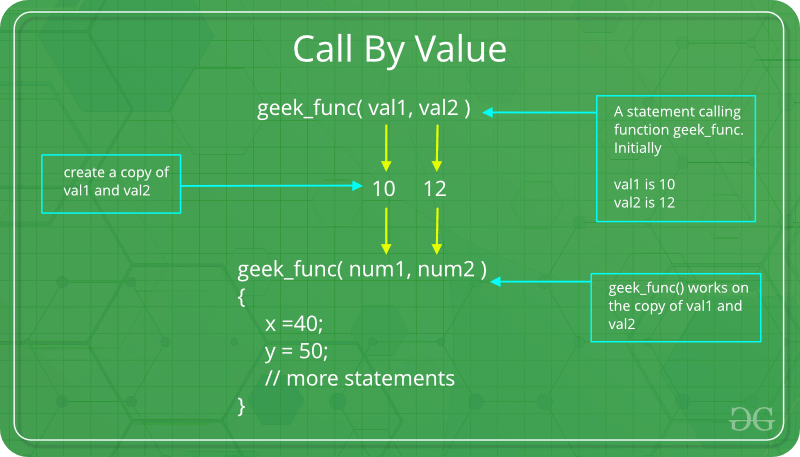`// C program to illustrate ` `// call by value ` `#include ` ` `  `void` `func(``int` `a, ``int` `b) ` `{ ` `    ``a += b; ` `    ``printf``(``"In func, a = %d b = %d\n"``, a, b); ` `} ` `int` `main(``void``) ` `{ ` `    ``int` `x = 5, y = 7; ` ` `  `    ``// Passing parameters ` `    ``func(x, y); ` `    ``printf``(``"In main, x = %d y = %d\n"``, x, y); ` `    ``return` `0; ` `} `

Output:

```In func, a = 12 b = 7
In main, x = 5 y = 7
```

Languages like C, C++, Java support this type of parameter passing. Java in fact is strictly call by value.
Shortcomings:

• Inefficiency in storage allocation
• For objects and arrays, the copy semantics are costly
2. Pass by reference(aliasing) : This technique uses in/out-mode semantics. Changes made to formal parameter do get transmitted back to the caller through parameter passing. Any changes to the formal parameter are reflected in the actual parameter in the calling environment as formal parameter receives a reference (or pointer) to the actual data. This method is also called as <em>call by reference. This method is efficient in both time and space.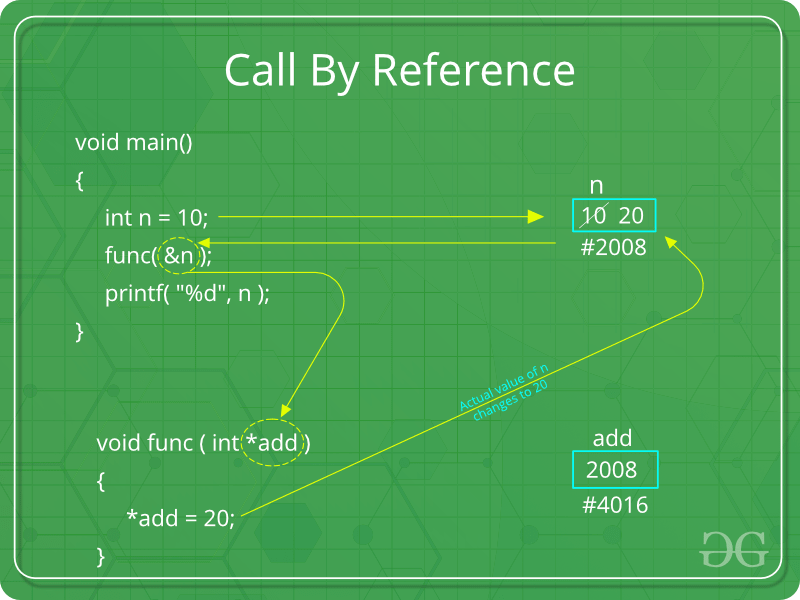`// C program to illustrate ` `// call by reference ` `#include ` ` `  `void` `swapnum(``int``* i, ``int``* j) ` `{ ` `    ``int` `temp = *i; ` `    ``*i = *j; ` `    ``*j = temp; ` `} ` ` `  `int` `main(``void``) ` `{ ` `    ``int` `a = 10, b = 20; ` ` `  `    ``// passing parameters ` `    ``swapnum(&a, &b); ` ` `  `    ``printf``(``"a is %d and b is %d\n"``, a, b); ` `    ``return` `0; ` `} `

Output:

```a is 20 and b is 10
```
3. Write a program in C to print the following pattern:

```ABCDEFGFEDCBA
ABCDEF FEDCBA
ABCDE   EDCBA
ABCD     DCBA
ABC       CBA
AB         BA
A           A```

 `#include ` ` `  `int` `main() ` `{ ` ` `  `    ``char` `ch = ``'A'``, ch1; ` `    ``int` `i, j, n = 7; ` ` `  `    ``for` `(``int` `i = 0; i < n; i++) { ` `        ``for` `(``int` `j = ``'A'``; j < ``'A'` `+ (2 * n) - 1; j++) { ` `            ``if` `(j >= (``'A'` `+ n - 1) + i) ` `                ``printf``(``"%c"``, ` `                       ``(``char``)((``'A'` `+ n - 1) ` `                              ``- (j % (``'A'` `+ n - 1)))); ` `            ``else` `if` `(j <= (``'A'` `+ n - 1) - i) ` `                ``printf``(``"%c"``, (``char``)j); ` `            ``else` `                ``printf``(``" "``); ` `        ``} ` `        ``printf``(``"\n"``); ` `    ``} ` ` `  `    ``return` `0; ` `} `

Output:

```ABCDEF FEDCBA
ABCDE   EDCBA
ABCD     DCBA
ABC       CBA
AB         BA
A           A
```
4. Write a program to check whether the given character is in upper case, lower case or non alphabetic character.

 `#include ` ` `  `// Driver Code ` `int` `main() ` `{ ` `    ``char` `ch; ` `    ``int` `ascii; ` ` `  `    ``// Get the character ` `    ``scanf``(``"%c"``, &ch); ` ` `  `    ``// Get ASCII value of the character ` `    ``ascii = ch; ` ` `  `    ``if` `(ascii >= 65 && ascii <= 91) ` `        ``printf``(``"%c is an UpperCase character"``, ch); ` ` `  `    ``else` `if` `(ascii >= 97 && ascii <= 122) ` `        ``printf``(``"%c is an LowerCase character"``, ch); ` ` `  `    ``else` `        ``printf``(``"%c is not an aplhabetic character"``, ch); ` ` `  `    ``return` `0; ` `} `

Output:

```A is an UpperCase character
a is an LowerCase character
0 is not an aplhabetic character
```

Here, a user can decide among multiple options. The if statements are executed from the top down. As soon as one of the conditions controlling the if is true, the statement associated with that if is executed, and the rest of the ladder is bypassed. If none of the conditions is true, then the final else statement will be executed.

Syntax:

```if (condition)
statement;
else if (condition)
statement;
.
.
else
statement;
```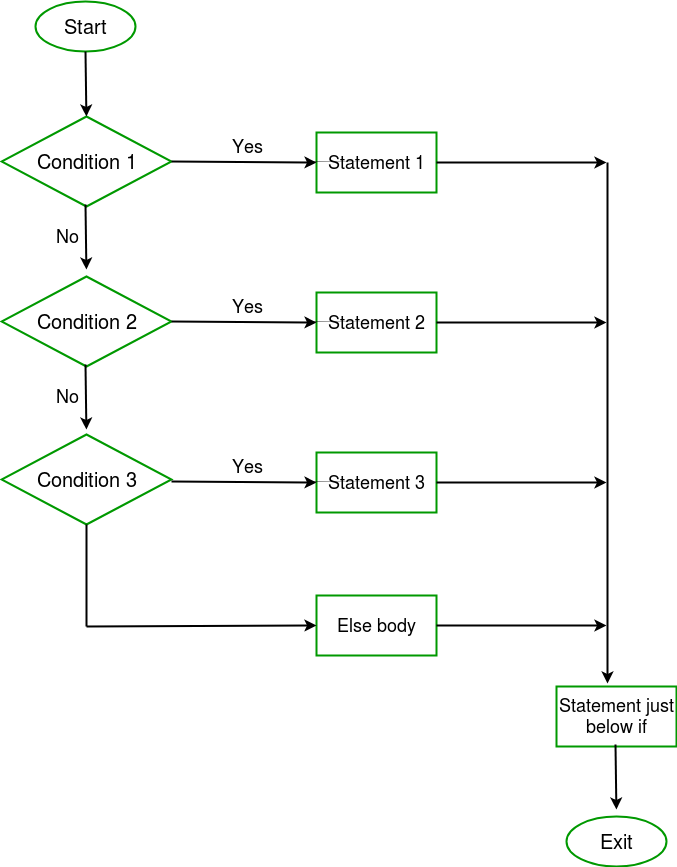Example:

 `// C++ program to illustrate if-else-if ladder ` ` `  `#include ` `using` `namespace` `std; ` ` `  `int` `main() ` `{ ` `    ``int` `i = 20; ` ` `  `    ``if` `(i == 10) ` `        ``cout << ``"i is 10"``; ` `    ``else` `if` `(i == 15) ` `        ``cout << ``"i is 15"``; ` `    ``else` `if` `(i == 20) ` `        ``cout << ``"i is 20"``; ` `    ``else` `        ``cout << ``"i is not present"``; ` `} `

Output:

```i is 20
```

• The if-else-if ladder are hard to understand and modify.
• As the length of if-else-if ladder increases, the readability of the program decreases.
• In situations involving a series of decisions one after the other, each decision or condition may involve expressions which result in integer value. In these situations, the usage of the if-else-if ladder is not recommended. Instead of this statement, we go for the switch statement.
6. What are the principles of recursion? Explain in detail.

The process in which a function calls itself directly or indirectly is called recursion and the corresponding function is called as recursive function. Using recursive algorithm, certain problems can be solved quite easily. Examples of such problems are Towers of Hanoi (TOH), Inorder/Preorder/Postorder Tree Traversals, DFS of Graph, etc.

What is base condition in recursion?
In recursive program, the solution to base case is provided and solution of bigger problem is expressed in terms of smaller problems.

```int fact(int n)
{
if (n < = 1) // base case
return 1;
else
return n*fact(n-1);
}
```

In the above example, base case for n < = 1 is defined and larger value of number can be solved by converting to smaller one till base case is reached.

How a particular problem is solved using recursion?
The idea is represent a problem in terms of one or more smaller problems, and add one or more base conditions that stop recursion. For example, we compute factorial n if we know factorial of (n-1). Base case for factorial would be n = 0. We return 1 when n = 0.

Program to find factorial of a number:

 `// C program to find factorial of given number ` `#include ` ` `  `// function to find factorial of given number ` `unsigned ``int` `factorial(unsigned ``int` `n) ` `{ ` ` `  `    ``// Base case ` `    ``if` `(n == 0) ` `        ``return` `1; ` ` `  `    ``// Recursively call factorial function ` `    ``return` `n * factorial(n - 1); ` `} ` ` `  `int` `main() ` `{ ` ` `  `    ``int` `num; ` ` `  `    ``// Ge the number of which ` `    ``// factorial is to be calculated ` `    ``scanf``(``"%d"``, &num); ` `    ``printf``(``"Enter the number: %d"``, num); ` ` `  `    ``// Find the factorial ` `    ``// and print the result ` `    ``printf``(``"\nFactorial of %d is %d"``, ` `           ``num, factorial(num)); ` ` `  `    ``return` `0; ` `} `

Output:

```Enter the number: 5
Factorial of 5 is 120```
7. Describe the relation between structures and pointers

Self Referential structures are those structures that have one or more pointers which point to the same type of structure, as their member.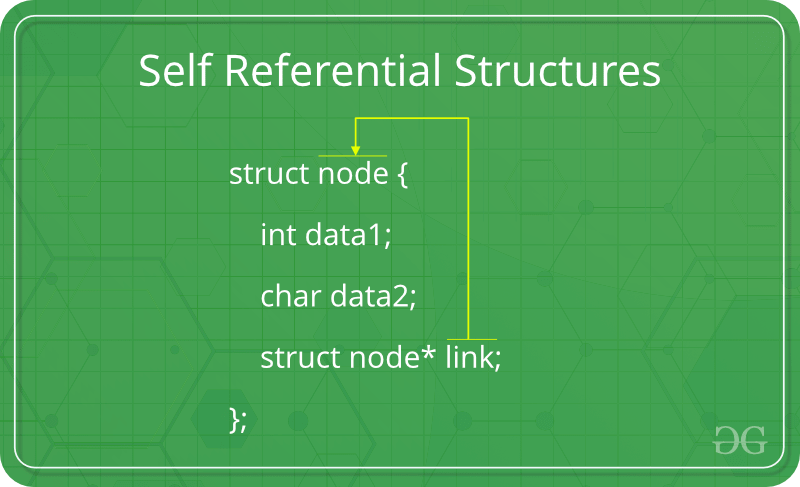In other words, structures pointing to the same type of structures are self-referential in nature.

Example:

 `struct` `node { ` `    ``int` `data1; ` `    ``char` `data2; ` `    ``struct` `node* link; ` `}; ` ` `  `int` `main() ` `{ ` `    ``struct` `node ob; ` `    ``return` `0; ` `} `

In the above example ‘link’ is a pointer to a structure of type ‘node’. Hence, the structure ‘node’ is a self-referential structure with ‘link’ as the referencing pointer.
An important point to consider is that the pointer should be initialized properly before accessing, as by default it contains garbage value.

Types of Self Referential Structures

1. Self Referential Structure with Single Link
2. Self Referential Structure with Multiple Links

Self Referential Structure with Single Link: These structures can have only one self-pointer as their member. The following example will show us how to connect the objects of a self-referential structure with the single link and access the corresponding data members. The connection formed is shown in the following figure.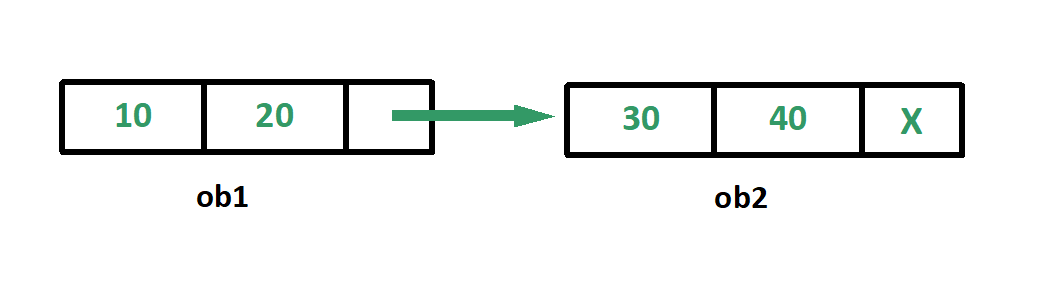`#include ` ` `  `struct` `node { ` `    ``int` `data1; ` `    ``char` `data2; ` `    ``struct` `node* link; ` `}; ` ` `  `int` `main() ` `{ ` `    ``struct` `node ob1; ``// Node1 ` ` `  `    ``// Intialization ` `    ``ob1.link = NULL; ` `    ``ob1.data1 = 10; ` `    ``ob1.data2 = 20; ` ` `  `    ``struct` `node ob2; ``// Node2 ` ` `  `    ``// Initialization ` `    ``ob2.link = NULL; ` `    ``ob2.data1 = 30; ` `    ``ob2.data2 = 40; ` ` `  `    ``// Linking ob1 and ob2 ` `    ``ob1.link = &ob2; ` ` `  `    ``// Accessing data members of  ob2 using ob1 ` `    ``printf``(``"%d"``, ob1.link->data1); ` `    ``printf``(``"\n%d"``, ob1.link->data2); ` `    ``return` `0; ` `} `

Output:

```30
40
```

Self Referential Structure with Multiple Links:
Self referential structures with multiple links can have more than one self-pointers. Many complicated data structures can be easily constructed using these structures. Such structures can easily connect to more than one nodes at a time. The following example shows one such structure with more than one links.

The connections made in the above example can be understood using the following figure.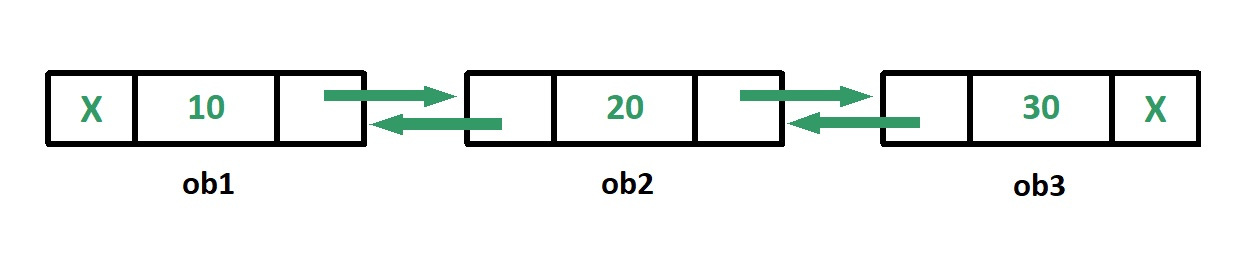`#include ` ` `  `struct` `node { ` `    ``int` `data; ` `    ``struct` `node* prev_link; ` `    ``struct` `node* next_link; ` `}; ` ` `  `int` `main() ` `{ ` `    ``struct` `node ob1; ``// Node1 ` ` `  `    ``// Intialization ` `    ``ob1.prev_link = NULL; ` `    ``ob1.next_link = NULL; ` `    ``ob1.data = 10; ` ` `  `    ``struct` `node ob2; ``// Node2 ` ` `  `    ``// Intialization ` `    ``ob2.prev_link = NULL; ` `    ``ob2.next_link = NULL; ` `    ``ob2.data = 20; ` ` `  `    ``struct` `node ob3; ``// Node3 ` ` `  `    ``// Intialization ` `    ``ob3.prev_link = NULL; ` `    ``ob3.next_link = NULL; ` `    ``ob3.data = 30; ` ` `  `    ``// Forward links ` `    ``ob1.next_link = &ob2; ` `    ``ob2.next_link = &ob3; ` ` `  `    ``// Backward links ` `    ``ob2.prev_link = &ob1; ` `    ``ob3.prev_link = &ob2; ` ` `  `    ``// Accessing  data of ob1, ob2 and ob3 by ob1 ` `    ``printf``(``"%d\t"``, ob1.data); ` `    ``printf``(``"%d\t"``, ob1.next_link->data); ` `    ``printf``(``"%d\n"``, ob1.next_link->next_link->data); ` ` `  `    ``// Accessing data of ob1, ob2 and ob3 by ob2 ` `    ``printf``(``"%d\t"``, ob2.prev_link->data); ` `    ``printf``(``"%d\t"``, ob2.data); ` `    ``printf``(``"%d\n"``, ob2.next_link->data); ` ` `  `    ``// Accessing data of ob1, ob2 and ob3 by ob3 ` `    ``printf``(``"%d\t"``, ob3.prev_link->prev_link->data); ` `    ``printf``(``"%d\t"``, ob3.prev_link->data); ` `    ``printf``(``"%d"``, ob3.data); ` `    ``return` `0; ` `} `

Output:

```10    20    30
10    20    30
10    20    30
```

In the above example we can see that ‘ob1’, ‘ob2’ and ‘ob3’ are three objects of the self referential structure ‘node’. And they are connected using their links in such a way that any of them can easily access each other’s data. This is the beauty of the self referential structures. The connections can be manipulated according to the requirements of the programmer.

Applications:
Self referential structures are very useful in creation of other complex data structures like:

8. What are the enumerated data type? Explain in detail.

Enumerated Data-type: Enumeration (or enum) is a user defined data type in C. It is mainly used to assign names to integral constants, the names make a program easy to read and maintain.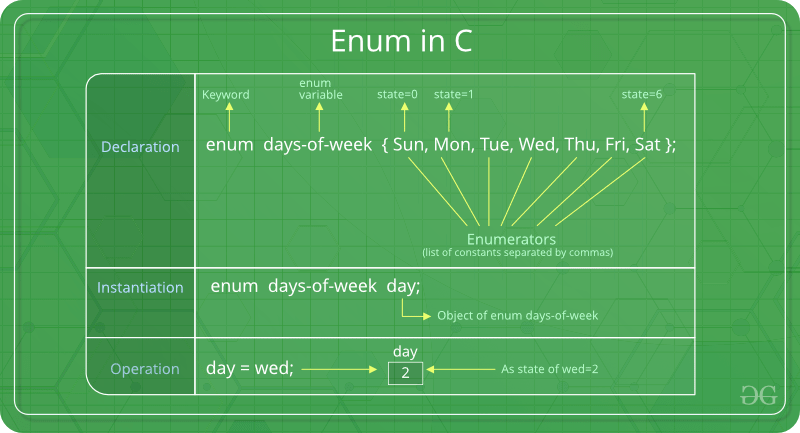`enum State {Working = 1, Failed = 0}; `

The keyword ‘enum’ is used to declare new enumeration types in C and C++.

Following is an example of enum declaration.

```// The name of enumeration is "flag" and the constant
// are the values of the flag. By default, the values
// of the constants are as follows:
// constant1 = 0, constant2 = 1, constant3 = 2 and
// so on.
enum flag{constant1, constant2, constant3, ....... };
```

Variables of type enum can also be defined. They can be defined in two ways:

```// In both of the below cases, "day" is
// defined as the variable of type week.

enum week{Mon, Tue, Wed};
enum week day;

// Or

enum week{Mon, Tue, Wed}day;
```

 `// An example program to demonstrate working ` `// of enum in C ` `#include ` ` `  `enum` `week { Mon, ` `            ``Tue, ` `            ``Wed, ` `            ``Thur, ` `            ``Fri, ` `            ``Sat, ` `            ``Sun }; ` ` `  `int` `main() ` `{ ` `    ``enum` `week day; ` `    ``day = Wed; ` `    ``printf``(``"%d"``, day); ` `    ``return` `0; ` `} `

Output:

```2
```

In the above example, we declared “day” as the variable and the value of “Wed” is allocated to day, which is 2. So as a result, 2 is printed.

Another example of enumeration is:

 `// Another example program to demonstrate working ` `// of enum in C ` `#include ` ` `  `enum` `year { Jan, ` `            ``Feb, ` `            ``Mar, ` `            ``Apr, ` `            ``May, ` `            ``Jun, ` `            ``Jul, ` `            ``Aug, ` `            ``Sep, ` `            ``Oct, ` `            ``Nov, ` `            ``Dec }; ` ` `  `int` `main() ` `{ ` `    ``int` `i; ` `    ``for` `(i = Jan; i <= Dec; i++) ` `        ``printf``(``"%d "``, i); ` ` `  `    ``return` `0; ` `} `

Output:

```0 1 2 3 4 5 6 7 8 9 10 11
```

In this example, the for loop will run from i = 0 to i = 11, as initially the value of i is Jan which is 0 and the value of Dec is 11.

My Personal Notes arrow_drop_upCheck out this Author's contributed articles.

If you like GeeksforGeeks and would like to contribute, you can also write an article using contribute.geeksforgeeks.org or mail your article to contribute@geeksforgeeks.org. See your article appearing on the GeeksforGeeks main page and help other Geeks.

Please Improve this article if you find anything incorrect by clicking on the "Improve Article" button below.

Article Tags :

Be the First to upvote.

Please write to us at contribute@geeksforgeeks.org to report any issue with the above content.